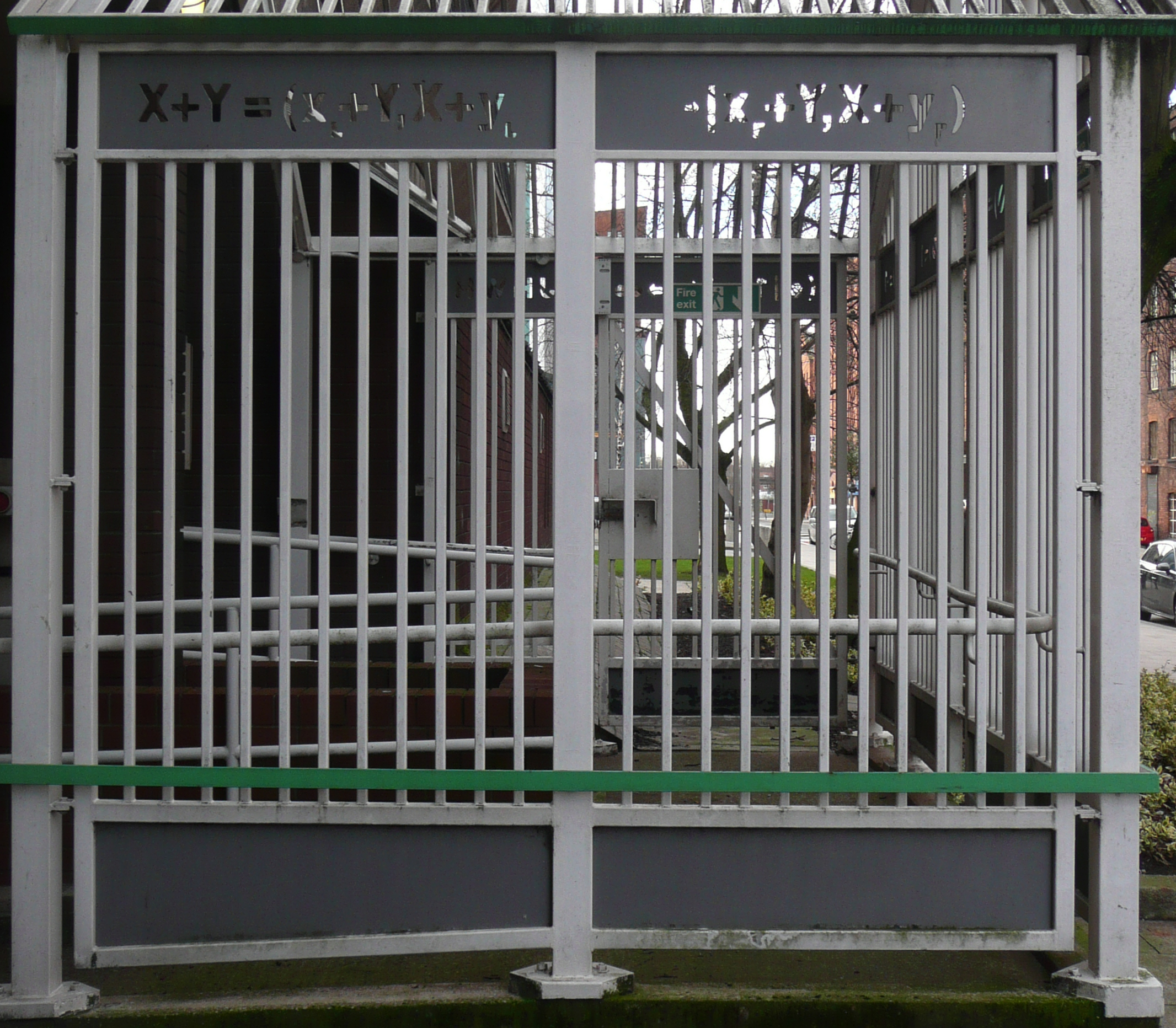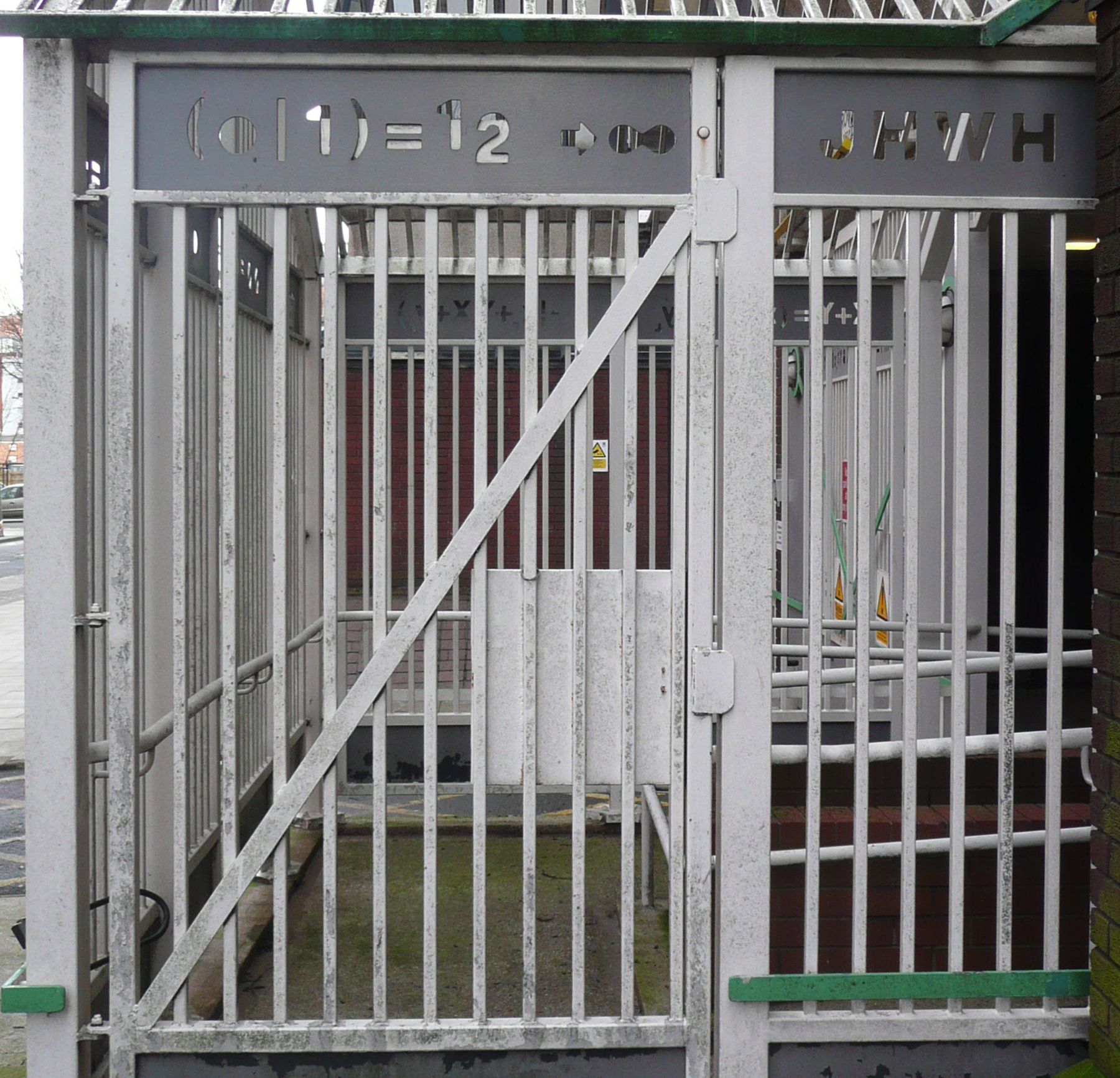Surreal goings-on at MMU

Surreal numbers are a construction developed by British mathematician John Conway (who also brought us the Game of Life). They are defined recursively: given two surreal numbers xL less than xR, we can define a new number X = (xL|xR) that bisects the two. Starting with the empty set and using this rule, we can generate all the real numbers, and more.

But, of course, those of you from Manchester knew all this already, as their definition may (surreally) be found on Chester Road, at the entrance to the MMU car park.$X = (x_L | x_R) {\rm ~iff~} \nexists x_R \leq x_L$$X \geq Y {\rm ~iff~} \nexists x_R \leq Y \& X \leq y_L$$X = Y {\rm ~iff~} X \leq Y \& Y \leq X$$X+Y=(x_L+Y,X+y_L | x_R+Y,X+y_R)$$\emptyset = {\rm void}$$(\emptyset,\emptyset) = 0$$(0, \emptyset) = 1$$(\emptyset, 0) = -1$$(0,1) = \frac{1}{2} \rightarrow \infty$${\rm JHWH}$

This final panel is my favourite. It seems to say that starting from nothing (∅) we can produce everything (∞),  thus proving the existence a higher power (JHWH, the Tetragrammaton). In fact it is being deliberately ambiguous; “JHWH” is also how American computer scientist Donald Knuth referred to Conway, writing in 1979:

In the beginning, everything was void, and J.H.W.H.Conway began to create numbers. Conway said, “Let there be two rules which bring forth all numbers large and small. This shall be the first rule: Every number corresponds to two sets of previously created numbers, such that no member of the left set is greater than or equal to any member of the right set. And the second rule shall be this: One number is less than or equal to another number if and only if no member of the first number’s left set is greater than or equal to the second number, and no member of the second number’s right set is less than or equal to the first number.” And Conway examined these two rules he had made, and behold! they were very good.

The mystery remains as to how this mathematical memorial found its way to such an unexpected location. Answers on a postcard.

Thanks to my collaborator and lunch-mate Jon Borresen for pointing out the gates.